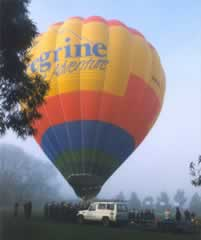Index Gases Absolute temperature Boyle's Law (PV = k) Carbon dioxide Charle's Law (V/T = k) Clouds and the gas laws. Combined gas law (PV/T = k) Fractional distillation of air Gas pressure (introduction) Hydrogen Introduction to gases Ideal gas Ideal gas constant (activity) Ideal gas equation Kinetic energy Kinetic theory Molar mass of carbon dioxide (activity) Oxygen Partial pressure Solubility in water Standard temperature, pressure S.T.PExercises - problem solving using the correct formula. Conversion of units.